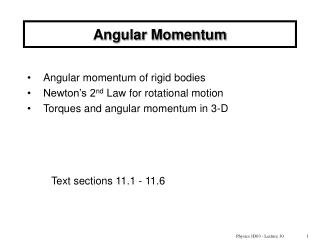DownloadDownload PresentationAngular Momentum

# Angular Momentum

Télécharger la présentation## Angular Momentum

- - - - - - - - - - - - - - - - - - - - - - - - - - - E N D - - - - - - - - - - - - - - - - - - - - - - - - - - -
##### Presentation Transcript

1. Angular Momentum • Angular momentum of rigid bodies • Newton’s 2nd Law for rotational motion • Torques and angular momentum in 3-D Text sections 11.1 - 11.6 Physics 1D03 - Lecture 30

2. mI vw Ft pL (“angular momentum”) “Angular momentum” is the rotational analogue of linear momentum. Recall linear momentum: for a particle, p = mv. Newton’s 2nd Law: The net external force on a particle is equal to the rate of change of its momentum. To get the corresponding angular relations for a rigid body, replace: Physics 1D03 - Lecture 30

3. (but not always). So, sometimes Angular momentum of a rotating rigid body: Angular momentum, L, is the product of the moment of inertia and the angular velocity. L = Iw Units: kg m2/s (no special name). Note similarity to: p=mv Newton’s 2nd Law for rotation: the torque due to external forces is equal to the rate of change of L. For a rigidbody (constant I ), Physics 1D03 - Lecture 30

4. Conservation of Angular momentum There are three great conservation laws in classical mechanics: • Conservation of Energy • Conservation of linear momentum • and now, Conservation of Angular momentum: In an isolated system (no external torques), the total angular momentum is constant. Physics 1D03 - Lecture 30

5. Angular Momentum Vector w For a symmetrical, rotating, rigid body, the vector L will be along the axis of rotation, parallel to the vectorw, and L = I w. L (In general L is not parallel to w, but Iw is still equal to the component of L along the rotation axis.) Physics 1D03 - Lecture 30

6. z L y O r v x f m Angular momentum of a particle This is the real definition of L. • L is a vector. • Like torque, it depends on the • choice of origin (or “pivot”). • If the particle motion is all in the x-y • plane, L is parallel to the z axis.. Physics 1D03 - Lecture 30

7. Angular momentum of a particle (2-D): |L| = mrvt = mvr sinθ r v m For a particle travelling in a circle (constant |r|, θ=90), vt = rw, so: L = mrvt = mr2w = Iw Physics 1D03 - Lecture 30

8. Quiz As a car travels forwards, the angular momentum vector L of one of its wheels points: • forwards • backwards E) left F) right C) up D) down Physics 1D03 - Lecture 30

9. Quiz A physicist is spinning at the center of a frictionless turntable, holding a heavy physics book in each hand with his arms outstretched. As he brings his arms in, what happens to the angular momentum? • increases • decreases • remains constant • What happens to the angular velocity? Physics 1D03 - Lecture 30

10. Example: A student sits on a rotating chair, holding two weights each of mass 3.0kg. When his arms are extended to 1.0m from the axis of rotation his angular speed is 0.75 rad/s. The students then pulls the weights horizontally inward to 0.3m from the axis of rotation.Given that I = 3.0 kg m2 for the student and chair, what is the new angular speed of the student ? Physics 1D03 - Lecture 30

11. w m2 v m1 v Example Angular momentum provides a neat approach to Atwood’s Machine. We will find the accelerations of the masses using “external torque = rate of change of L”. O R Physics 1D03 - Lecture 30

12. O R R r w p1 p1 m2 v m1 v Atwoods Machine, frictionless (at pivot), massive pulley For m1 :L1 = |r1x p1|= Rp1 so L1= m1vR L2= m2vR Lpulley= Iw = Iv/R Thus L = (m1 +m2 + I/R2)v R so dL/dt = (m1 +m2 + I/R2)a R Torque, t = m1gR- m2gR = (m1 - m2 )gR Write t = dL/dt, and complete the calculation to solve for a. Note that we only consider the external torques on the entire system. Physics 1D03 - Lecture 30

13. Solution Physics 1D03 - Lecture 30

14. Summary Particle: Any collection of particles: L = I w. Rotating rigid body: Newton’s 2nd Law for rotation: Angular momentum is conserved if there is no external torque. Physics 1D03 - Lecture 30